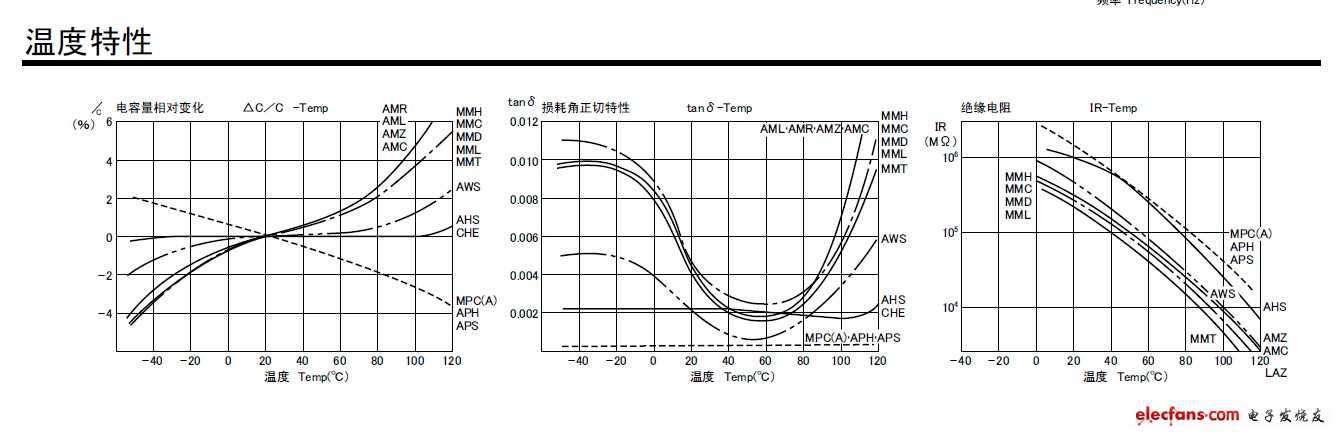» » » What is the temperature characteristic of a capacitor?

# What is the temperature characteristic of a capacitor?

Views:0     Author:Site Editor     Publish Time: 2020-09-07      Origin:Site1. Temperature and life of capacitor. Usually the life of the capacitor decreases with the increase in temperature, the most obvious: electrolytic capacitor. An electrolytic capacitor with a limit working temperature of 85°C can generally guarantee a normal working time of 181019 hours when working at 20°C; and when working at a limit temperature of 85°C, it can generally only guarantee a normal working time of 2000 hours. operating hours. So pay attention to this situation when designing the circuit.

2. The tangent of the loss angle between temperature and capacitance. Any capacitor has a loss tangent value, that is, the loss of the capacitor. Generally, the tangent value increases with increasing temperature. For example, the loss tangent value of the CC10 ultra-high frequency ceramic dielectric capacitor is 0.0012 at normal temperature (20℃±5℃), but it is 0.0018 at the positive limit temperature (85℃). It can be seen that it is greatly affected by temperature.

3. Insulation resistance between temperature and capacitance. Generally, the insulation resistance of a capacitor decreases with the increase of temperature, and the decrease of the insulation resistance will cause the leakage current of the capacitor to increase.

4. Temperature and capacitance of capacitor. The temperature coefficient of a capacitor means that the capacitance of a capacitor changes with temperature. When designing a precision capacitor timing circuit and an oscillating circuit whose frequency is determined by the capacitor, the impact of temperature on the capacitance of the capacitor should be fully considered. Otherwise, the designed capacitor timing circuit will have inaccurate timing; the oscillation frequency of the oscillation circuit will vary with temperature The changes change.

In short, when using a capacitor, the effect of temperature on the capacitance should be fully considered, and try to make the capacitor work at about 20°C.

Capacitance temperature coefficient Capacitance temperature coefficient is divided into class I and class II. Class I capacitors are divided into 8 levels, and class II capacitors are divided into 5 levels.